Tamilnadu State Board New Syllabus Samacheer Kalvi 11th Maths Guide Pdf Chapter 4 Combinatorics and Mathematical Induction Ex 4.1 Text Book Back Questions and Answers, Notes.

## Tamilnadu Samacheer Kalvi 11th Maths Solutions Chapter 4 Combinatorics and Mathematical Induction Ex 4.1

Question 1.
(i) A person went to a restaurant for dinner. In the menu card, the person saw 10 Indian and 7 Chinese food items. In how many ways the person can select either an Indian or Chinese food?
Selecting an Indian food item from the given 10 can be done in 10 ways. Selecting a Chinese food item from the given 7 can be done in 7 ways.
∴ Selecting an Indian or Chinese food can be done in 10 + 7 = 17 ways.(ii) There are 3 types of a toy car and 2 types of toy train are available in a shop. Find the number of ways a baby can buy a toy car and a toy train?
Number of types of Toy car = 3
Number of types of Toy Train = 2
Number of ways of buying a Toy car = 3 ways
Number of ways of buying a toy train = 2 ways
∴ By fundamental principle of multiplication, number of ways of buying a toy car and a toy train = 3 × 2 ways = 6 ways

(iii) How many two – digit numbers can be formed using 1 , 2,3,4,5 without repetition of digits?The given digits are 1, 2, 3, 4, 5
A two-digit number has a unit place and 10’s place. We are given 5 digits (1, 2, 3, 4, 5). The unit place can be filled (using the 5 digits) in 5 ways. After filling the unit place since repetition is not allowed one number (filled in the unit place) should be excluded. So the 10’s place can be filled (using the remaining 4 digits) in 4 ways.
∴ Unit place and 10’s place together can be filled in 5 × 4 = 20 ways. So the number of two-digit numbers = 20

(iv) Three persons enter into a conference hall in which there are 10 seats. In how many ways can they take their seats?
Number of seats in the conference hall = 10
Number of persons entering into the conference hall = 3
Number of ways of getting a seat for 1st person = 10
Number of ways of getting a seat for 2nd person = 9
Number of ways of getting a seat for 3rd person = 8
By fundamental principle of multiplication, number of ways of getting seats for 3 persons in conference hall = 10 × 9 × 8 ways = 720 ways(v) In how ways 5 persons can be seated In a row?
Number of persons = 5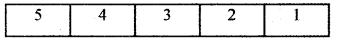5 persons can be arranged among themselves in 5! ways
(i.e) 5 × 4 × 3 × 2 × 1 = 120 ways

Question 2.
(i) A mobile phone has a passcode of 6 distinct digits. What is the maximum number of attempts one makes to retrieve the passcode?
Number of distinct digit in a passcode of a mobile phone = 6First digit can be tried in 10 ways using the digits 0, 1, 2, 3, 4, 5, 6, 7, 8, 9
Second digit can be tried in 9 ways
Third digit can be tried in 8 ways
Fourth digit can be tried in 7 ways
Fifth digit can be tried in 6 ways
Sixth digit can be tried in 5 ways
Therefore, the maximum number of attempts made to retrieve the passcode = 10 × 9 × 8 × 7 × 6 × 5 = 151200(ii) Given four flags of different colours, how many different signals can be generated if each signal requires the use of three flags, one below the other?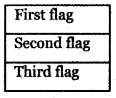Number of flags = 4
Number of flags required for a signal = 3
The total number of signals is equal to the number of ways of filling 3 places in succession by 4 flags of different colours. Number of ways of filling the top place using 4 different colour flags is 4 ways. Number of ways of filling the middle place using the remaining 3 different colour flags is 3 ways. Number of ways of filling the bottom place using the remaining 2 different colour flags is 2 ways.

Therefore, by fundamental principle of multiplication, the total number of signals = 4 × 3 × 2 = 24 ways

Question 3.
Four children are running a race.
(i) In how many ways can the first two places be filled?
(ii) In how many different ways could they finish the race?
(i) Number of children in the running race = 4
The first place can be filled in (from the 4 children) 4 ways
After filling in the first place only 3 children are left out
So the second place can be filled in (from the remaining 3 children) 3 ways
So the first and the second places together can be filled in 4 × 3 = 12 ways

(ii) The first and second places can be filled in 12 ways
The third-place can be filled (from the remaining 2 children) in 2 ways and the fourth place can be filled in 1 way
So the race can be finished in 12 × 2 × 1 = 24 waysQuestion 4.
Count the number of three – digit numbers which can be formed from the digits 2, 4, 6, 8 if
(i) Repetitions of digits is allowed.
(ii) Repetitions of digits is not allowed?
(i) Repetitions of digits is allowed
The given digits are 2, 4, 6, 8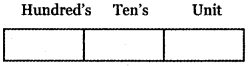A number of ways of filling the unit place using the 4 digits 2, 4, 6, 8 is 4 ways. Number of ways of filling the tens place using the 4 digits 2, 4, 6, 8 in 4 ways Number of ways of filling the hundred’s place using the 4 digits 2, 4, 6, 8 is 4 ways

Therefore, by fundamental principle of multiplication, the total number of 3 digit numbers = 4 × 4 × 4
= 64 ways(ii) The unit place can be filled (using the 4 digits) in 4 ways after filling the unit place since repetition of digits is not allowed that digit should be excluded.
So the 10’s place can be filled in (4 – 1) 3 ways and the 100’s place can be filled in (3 – 1) 2 ways
So the unit place, 10’s and 100’s places together can be filled in 4 × 3 × 2 = 24 ways
(i.e) The number of 3 digit numbers = 4 × 3 × 2 = 24 ways

Question 5.
How many three-digit numbers are there with 3 in the unit place?
(i) with repetition
(ii) without repetition.
(i) With repetition: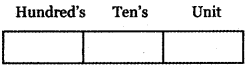The given digits are 0, 1, 2, 3, 4, 5, 6, 7, 8, 9
The unit place can be filled in only one way using the digit 3. The ten’s place can be filled in 10 ways using the digits 0, 1, 2, 3, 4, 5, 6, 7, 8, 9. The hundred’s place can be filled in 9 ways using the digits 1, 2, 3, 4, 5, 6, 7, 8, 9.

Therefore, by the fundamental principle of multiplication, the total number of 3 digit numbers is = 1 × 10 × 9 = 90

(ii) Without repetition: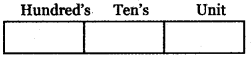The digits are 0, 1,2, 3, 4, 5, 6, 7, 8, 9
A three-digit number has 3 digits l’s, 10’s, and 100’s place.
The unit place is (filled by 3) filled in one way.
After filling the unit place since the digit ‘0’ is there, we have to fill the 100’s place. Now to fill the 100’s place we have 8 digits (excluding 0 and 3) So 100’s place can be filled in 8 ways.
Now to fill the 10’s place we have again 8 digits (excluding 3 and any one of the number) So 10’s place can be filled in 8 ways.
∴ Number of 3 digit numbers with ‘3’ in unit place = 8 × 8 × 1 = 64Question 6.
How many numbers are there between 100 and 500 with the digits 0,1,2,3,4,5 if
(i) Repetition of digit is allowed
(ii) Repetition of digits is not allowed.
(i) Repetition of digit is allowed:The numbers between 100 and 500 will have 3 digits. The unit place can be filled in 6 ways using the digits 0, 1, 2, 3, 4, 5. The ten’s place can be filled in 6 ways using the digits 0, 1, 2, 3, 4, 5. The hundred’s place can be filled in 4 ways using the digits 0, 1, 2, 3, 4, 5 ( Excluding 0 and 5). Therefore, by fundamental principle of multiplication, the number of 3 digit, numbers between 100 and 500 with repetition of digits using the digits 0, 1, 2, 3, 4, 5 is = 6 × 6 × 4 = 144

(ii) Repetition of digits is not allowed: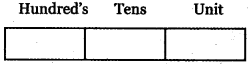The 100’s place can be filled (by using 1, 2, 3, 4) 10’s in 4 ways
The 10’s place can be filled in (6 – 1) 5 ways and the unit place can be filled in (5 – 1) 4 ways
So the number of 3 digit number 4 × 5 × 4 = 80

Question 7.
How many three-digit odd numbers can be formed by using the digits 0, 1, 2, 3, 4, 5? if
(i) The repetition of digits is not allowed
(ii) The repetition of digits is allowed.
The given digits are 0, 1, 2, 3, 4, 5
To find the possible 3 – digit odd numbers.

(i) Repetition of digits is not allowed:Since we need 3 – digit odd numbers the unit place can be filled in 3 ways using the digits 1, 3 or 5. Hundred’s place can be filled in 4 ways using the digits 0, 1, 2, 3, 4, 5 excluding 0 and the number placed in unit place. Ten’s place can be filled in 4 ways using the digits 0, 1, 2, 3, 4, 5 excluding the digit placed in the hundred’s place.

Therefore, by the fundamental principle of multiplication, the number of 3 – digit odd numbers formed without repetition of digits using the digits 0, 1, 2, 3, 4, 5 is
= 4 × 4 × 3 = 48(ii) Repetition of digits is allowed: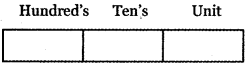The unit place can be filled in 3 ways. We are given 6 digits.
So 10’s place can be filled in 6 ways and the 100’s place can be filled in (6 – 1) (excluding zero) 5 ways
So the Number of 3 digit numbers = 3 × 6 × 5 = 90

Question 8.
Count the numbers between 999 and 10000 subject to the condition that there are
(i) no restriction
(ii) no digit is repeated
(iii) At atleast one of the digits is repeated.
To find the numbers between 999 and 10,000 using the digits 0, 1, 2, 3, 4, 5, 6, 7, 8, 9
To find the possible 4 digit numbers.

(i) No restriction:Thousand’s place can be filled in 9 ways using the digits 0, 1, 2, 3, 4, 5, 6, 7, 8, 9 excluding 0. Since there is no restriction, the hundred’s place, Ten’s place, and the unit place can be filled in 10 ways using the digit 0, 1, 2, 3, 4, 5, 6, 7, 8, 9.

Therefore, by the fundamental principle of multiplication, the number of 4 digit numbers between 999 and 10,000 is = 9 × 10 × 10 × 10 = 9000

(ii) No digit is repeated:Thousand’s place can be filled in 9 ways using the digits 0, 1, 2, 3, 4, 5, 6, 7, 8, 9 excluding 0. Since repetition is not allowed. The unit place can be filled in 9 ways using the digits 0,1, 2, 3, 4, 5, 6, 7, 8, 9 excluding the digit placed in the thousand’s place. Since repetition of digits is not allowed, the ten’s place can be filled in 8 ways using the digits 0, 1, 2, 3, 4, 5, 6, 7, 8, 9 excluding the digits used in thousand’s a place and unit place. Since repetition of digits is not allowed, the hundred’s place can be filled in 7 ways using the digits 0,1,2,3,4, 5,6,7,8,9 excluding the digits used in thousand’s a place and unit place.

Therefore, by the fundamental principle of multiplication, the number of numbers between 999 and 10,000 without repetition of digits is = 9 × 7 × 8 × 9 = 4536(iii) At least one of the digits is repeated:
Required number of 4 digit numbers = Total number of 4 digit numbers – Number of 4 digit numbers when no digit is repeated = 9000 – 4536 = 4464

Question 9.
How many three-digit numbers, which are divisible by 5, can be formed using the digits 0, 1, 2, 3, 4, 5 if
(i) Repetition of digits is not allowed?
(ii) Repetition of digits is allowed?
The given digits are 0, 1, 2, 3, 4, 5
To find the 3 – digit numbers formed by using the digits 0, 1, 2, 3, 4, 5 which are divisible by 5.

(i)The repetition of digits are not allowed: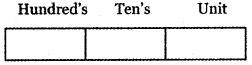Since the 3 – digit number is divisible by 5, the unit place can be filled in 2 ways using the digits 0 or 5

Case (i) When the unit place is filled with the digit 0. The hundreds place can be filled in 5 ways using the digits 1, 2, 3, 4, 5 and the ten’s place can be filled in 4 ways using the digits 0, 1, 2, 3, 4, 5 excluding 0 and the digit which is placed in hundred’s place.

Therefore, by fundamental principle of multiplication, the number of 3 – digit numbers divisible by 5 is
= 5 × 4 × 1 = 20

Case (ii) When the unit place is tilled with the digit 5, since repetition of digit is not allowed the hundred’s place can be filled in 4 ways using the digits 1, 2 , 3 , 4 (0 and 5 are excluded). The ten’s place can be filled in 4 ways using the digits 0, 1 , 2, 3 , 4, 5 (excluding 5 and the digit placed in the hundred’s place).
Therefore, by the fundamental principle of multiplication, the number of 3 – digit numbers, in this case, is = 4 × 4 × 1 = 16
Therefore, the total number of 3 – digit numbers divisible by 5 using the digits 0, 1, 2, 3, 4, 5 is = 20 + 16 = 36(ii) The repetition of digits are allowed:The digits are
0 1 2 3 4 5
To get a number divisible by 5 we should have the unit place as 5 or 0 So the unit place (using 0 or 5) can be filled in 2 ways.
The 10’s place can be filled (Using 0, 1, 2, 3, 4, 5) in 6 ways and the 100’s place (Using 1, 2, 3, 4, 5) can be filled in 5 ways.
So the number of 3 digit numbers ÷ by 5 (with repetition) = 2 × 6 × 5 = 60

Question 10.
To travel from place A to place B, there are two different bus routes B1, B2, two different train routes T1, T2, and one air route A1. From place B to place C, there is one bus route say B’1, two different train routes say T’1, T’2, and one air route A’1. Find the number of routes of commuting from place A to place C via place B without using a similar mode of transportation.
Route map diagram for the given data.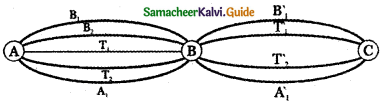The possible choices for a number of routes commuting from A to place C via place B without using similar mode transportation are
(B1, T’1), (B1, T’2), ( B1, A1), ( B2, T’1), (B2, T’2)
(B2, A’1), (T1, B’1), (T1, A’1), ( T2, B’1), ( T2, A’1) (A1, B’1) , (A1, T’1) and (A1, T’2)
Therefore, the Required number of routes is 13.

Question 11.
How many numbers are there between 1 and 1000 (both inclusive) which are divisible neither by 2 nor by 5 ?
From 1 to 1000, the numbers ÷ by 2 = 500
the number ÷ by 5 = 200
and the numbers ÷ by 10 = 100(5 × 2 = 10)
So number ÷ by 2 or 5 = 500 + 200 – 100 = 600
Total numbers from 1 to 1000 = 1000
So the number of numbers which are ÷ neither by 2 nor by 5 = 1000 – 600 = 400

Three-digit numbers: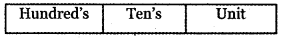The unit place can be filed in 4 ways using the digits 1, 3, 7, 9. Hundred’s place can be filled in 9 ways excluding 0. Ten’s place can be filled in 10 ways using the digits 0, 1, 2, 3, 4, 5, 6, 7, 8, 9. Therefore, the required number of 3 digit numbers neither divisible by 2 nor by 5 is = 9 × 10 × 4 = 360.
There is only one 4 – digit number, but it is divisible by 2 and 5.
Therefore, required numbers using fundamental principle of addition = 4 + 36 + 360 = 400Question 12.
How many strings can be formed using the letters of the word LOTUS if the word
(ii) neither starts with L nor ends with S?
(i) Either starts with L or ends with S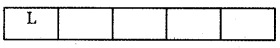The first box is filled with the letter L. The second box can be filled with the remaining letters O, T, U, S in 4 ways. The third box can be filled with the remaining letters excluding L and the letter placed in box 2 in 3 ways. The fourth box can be filled with the remaining letters excluding L and the letters placed in a box – 2 and box – 3 in 2 ways. The fifth box can be filled with the remaining one letter excluding L and the letters placed in a box – 2 and box – 3, box – 4 in 1 way.
Therefore, by fundamental principle of multiplication, the number of words start with L is = 1 × 4 × 3 × 2 × 1 = 24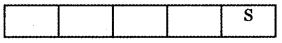Since the word ends with S, the fifth box can be filled in one way with the letter S. The remaining four boxes can be filled 4 × 3 × 2 × 1 way.
Therefore, the number of words ending with S = 4 × 3 × 2 × 1 × 1 = 24

Number of words starting with L and ends with S: The first box can be filled with L in one way fifth box can be filled with S in one way second box, third box, and fourth box can be filled in 3x2x1 ways with the remaining letters O, T, U.
∴ Number of words starting with L and ends with S = 1 × 3 × 2 × 1 × 1 = 6
Therefore, by fundamental principle of addition, number of words either starts with L or ends with S = 24 + 24 – 6 = 48 – 6 = 42

(ii) Neither starts with L nor ends with S Total number of words formed using the letters L, O, T, U, S is = 5 × 4 × 3 × 2 × 1 = 120
The number of words neither starts with L nor ends with S = Total number of words – Number of words starts with either L or ends with S = 120 – 42 = 78MCQ Questions For Class 11 hindi with answers·

Question 13.
(i) Count the total number of ways of answering 6 objective type questions, each question having 4 choices.
Count the total number of ways of answering 6 objective type questions, each question having 4 choices.
One question can be answered in 4 ways
Two questions can be answered in 4 × 4 = 42 ways
∴ Six questions can be answered in 46 ways

(ii) In how many ways 10 pigeons can be placed in 3 different pigeon holes?
Solution:
First pigeons can be placed in a pigeon-hole in 3 ways (selecting 1 from 3 holes)
Second pigeons can be placed in a pigeon-hole in 3 ways Tenth pigeons can be placed in a pigeon-hole in 3 ways
So total number of ways in which all the number 10 place can be sent = 3 × 3 × 3 × 3 × 3 × 3 × 3 × 3 × 3 × 3 = 310 ways

(iii) Find the number of ways of distributing 12 distinct prizes to 10 students?
Solution:
To give the first prize we have to select, from the 10 students which can be done in 10 ways.
To give the second prize we have to select one from the 10 students which can be done is 10 ways.
To give the 12th prize we have to select one from 10 students which can be done in 10 ways.
So all the 12 prizes can be given in (10 × 10 × 10 …. 12 times) = 1012 ways.

Question 14.
Find the value of
(i) 6!
(ii) 4! +5!
(iii) 3! – 2!
(iv) 31 × 21
(v) $$\frac{12 !}{9 ! \times 3 !}$$
(vi) $$\frac{(n+3) !}{(n+1) !}$$
(i) 6!
6! = 6 × 5 × 4 × 3 × 2 × 1 = 720

(ii) 4! +5!
4! +5! = (4 × 3 × 2 × 1) + (5 × 4 × 3 × 2 × 1)
= (4 × 3 × 2 × 1) [1 + 5]
= 24 × 6 = 144

(iii) 3! – 2!
3! – 2! = (3 × 2 × 1) × (4 × 3 × 2 × 1)
= 6 × 24 = 144

(iv) 3! × 2!
3! × 2! = (3 × 2 × 1) × (4 × 3 × 2 × 1)
= 6 × 24 = 144

(v) $$\frac{12 !}{9 ! \times 3 !}$$= 2 × 11 × 10 = 220

(vi) $$\frac{(n+3) !}{(n+1) !}$$= (n + 3) (n + 2)
= n2 + 3n + 2n + 6
= n2 + 5n + 6Question 15.
Evaluate $$\frac{\mathbf{n} !}{\mathbf{r} !(\mathbf{n}-\mathbf{r}) !}$$ when
(i) n = 6 , r = 2
(ii) n = 10, r = 3
(iii) For any n with r = 2.
(i) n = 6 , r = 2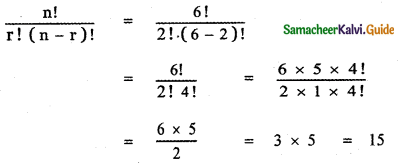(ii) n = 10, r = 3(iii) For any n with r = 2.Question 16.
Find the value of n if
(i) ( n + 1) ! = 20 ( n – 1 )!
(ii) $$\frac{1}{8 !}+\frac{1}{9 !}=\frac{n}{10 !}$$
(ii) $$\frac{1}{8 !}+\frac{1}{9 !}=\frac{n}{10 !}$$